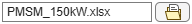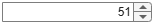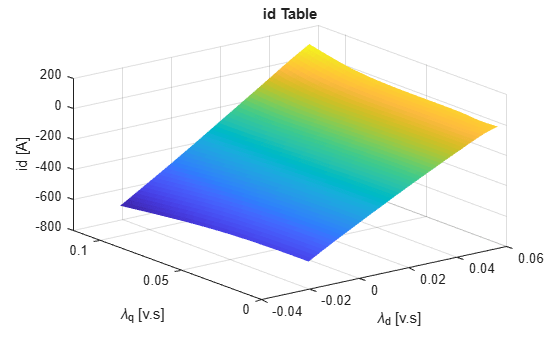# Generate Parameters for Flux-Based PMSM Block

This example shows how to create lookup tables for an interior permanent magnet synchronous motor (PMSM) controller that characterizes the d-axis and q-axis current as a function of d-axis and q-axis flux.

To generate the flux parameters for the Flux-Based PMSM block, follow these workflow steps.

### Step 1: Load and Preprocess Data

Load and preprocess this nonlinear motor flux data from dynamometer testing or finite element analysis (FEA):

• d- and q- axis current

• d- and q- axis flux

• Electromagnetic motor torque

Import and preprocess the raw PMSM data from a |xlsx| file captured from a dynamometer or another CAE tool.

fileName ="PMSM_150kW.xlsx"; opts = detectImportOptions(fileName); % read units if they are defined in the second row if opts.DataRange=="A3" opts.VariableUnitsRange = "A2"; end rawData = readtable(fileName, opts); 

lambda_d = rawData.Psi_d; lambda_q = rawData.Psi_q; id = rawData.I_d; iq = rawData.I_q;

Determine the minimum and maximum flux values.

flux_d_min = min(lambda_d); flux_d_max = max(lambda_d); flux_q_min = min(lambda_q); flux_q_max = max(lambda_q);

### Step 2: Generate Evenly Spaced Table Data From Scattered Data

The flux tables and can have different step sizes for the currents. Evenly spacing the rows and columns helps improve interpolation accuracy. This script uses spline interpolation.

Set the spacing for the table rows and columns.

flux_d_size =51; flux_q_size =51;

Generate linear spaced vectors for the breakpoints.

ParamFluxDIndex = linspace(flux_d_min,flux_d_max,flux_d_size); ParamFluxQIndex = linspace(flux_q_min,flux_q_max,flux_q_size); fluxBreakpoints = {ParamFluxDIndex, ParamFluxQIndex};

#### Fit the Id and Iq Lookup Tables

Create the table for the d-axis current. The d-axis current, Id, is a function of q-axis flux, λq, and d-axis flux, λd.

id_fit = fitlookupn(fluxBreakpoints,[lambda_d,lambda_q],id); ParamIdLookupTable = id_fit; figure; surf(ParamFluxDIndex,ParamFluxQIndex,ParamIdLookupTable'); xlabel('\lambda_d [v.s]');ylabel('\lambda_q [v.s]');zlabel('id [A]');title('id Table'); grid on; shading flat;Create the table for the q-axis current. The q-axis current, Iq, is a function of q-axis flux, λq, and d-axis flux, λd.

iq_fit = fitlookupn(fluxBreakpoints,[lambda_d,lambda_q],iq); ParamIqLookupTable = iq_fit; figure; surf(ParamFluxDIndex,ParamFluxQIndex,ParamIqLookupTable'); xlabel('\lambda_d [v.s]');ylabel('\lambda_q [v.s]');zlabel('iq [A]'); title('iq Table'); grid on; shading flat;### Step 3: Set Block Parameters

Set these Flux-Based PMSM block parameters to the values assigned in the example script.

Vector of d-axis flux, flux_d

flux_d=ParamFluxDIndex;

Vector of q-axis flux, flux_q

flux_q=ParamFluxQIndex;

Corresponding d-axis current, id

id=ParamIdLookupTable;

Corresponding q-axis current, iq

iq=ParamIqLookupTable;

 Hu, Dakai, Yazan Alsmadi, and Longya Xu. “High-Fidelity Nonlinear IPM Modeling Based on Measured Stator Winding Flux Linkage.” IEEE® Transactions on Industry Applications 51, no. 4 (July/August 2015).

 Chen, Xiao, Jiabin Wang, Bhaskar Sen, Panagiotis Lasari, and Tianfu Sun. “A High-Fidelity and Computationally Efficient Model for Interior Permanent-Magnet Machines Considering the Magnetic Saturation, Spatial Harmonics, and Iron Loss Effect.” IEEE Transactions on Industrial Electronics 62, no. 7 (July 2015).

 Ottosson, J., and M. Alakula. “A Compact Field Awakening Controller Implementation.” International Symposium on Power Electronics, Electrical Drives, Automation and Motion, July 2006.